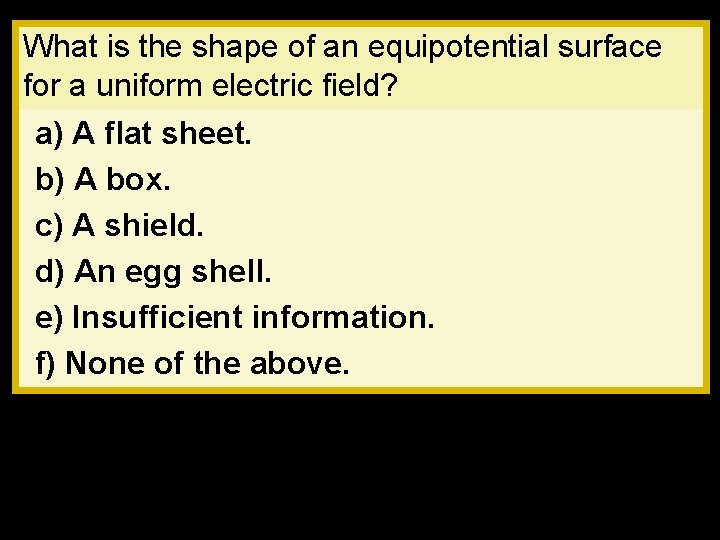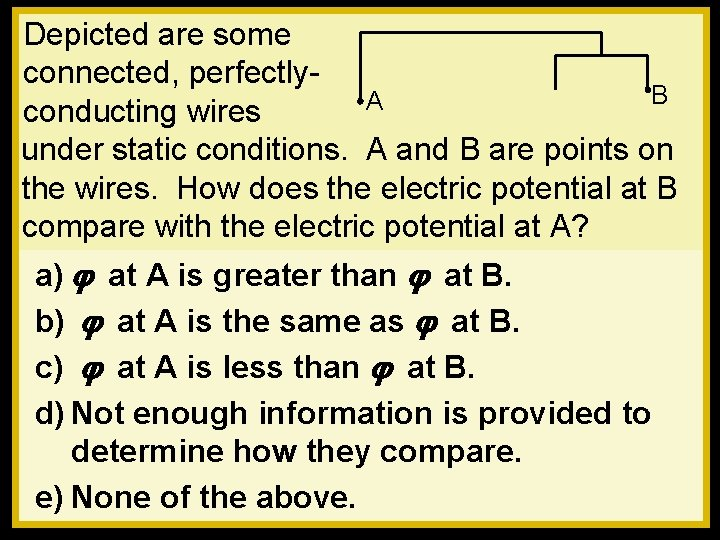# What is the shape of an equipotential surface

• Slides: 4What is the shape of an equipotential surface for a uniform electric field? a) A flat sheet. b) A box. c) A shield. d) An egg shell. e) Insufficient information. f) None of the above.Depicted are some connected, perfectly. B A conducting wires under static conditions. A and B are points on the wires. How does the electric potential at B compare with the electric potential at A? a) j at A is greater than j at B. b) j at A is the same as j at B. c) j at A is less than j at B. d) Not enough information is provided to determine how they compare. e) None of the above.Depicted are two sets of connected perfectly. C conducting wires under B D static conditions. The A potential at point B is 6 volts greater than the potential at point A. A particle of charge 1 e is caused to move from point C to point D. What is the change in the electric potential energy of the particle? a) – 6 e. V b) 0 c) +6 e. V d) None of the above because the e. V is not a unit of energy.Demo: I have a Ping-Pong ball and a rubber ball that is about the size of a baseball, each coated with conducting paint. I use a high voltage power supply to cause each, in relative isolation, to have a potential of 2400 volts. At that potential, which has more charge on it? a) The Ping-Pong ball. b) The rubber ball. c) Neither.